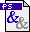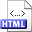A randomized in-place algorithm for positioning the kth element in a multiset Authors: Jyrki Katajainen and Tomi A. Pasanen Published in: Proceedings of the 8th Scandinavian Workshop on Algorithm Theory, Lecture Notes in Computer Science 2368, Springer-Verlag (2002), 408-417 Full text:PS (723 kB) DOI: 10.1007/3-540-45471-3_42 Copyright: © Springer-Verlag Abstract: A variant of the classical selection problem, called the positioning problem, is considered. In this problem we are given a sequence A[1∶n] of size n, an integer k, 1 ≤ k ≤ n, and an ordering function  ◯< , and the task is to rearrange the elements of the sequence such that A[k]  ◯<  A[j] is false for all j, 1 ≤ j < k, and A[ℓ]  ◯<  A[k] is false for all ℓ, k < ℓ ≤ n. We present a Las-Vegas algorithm which carries out this rearrangement efficiently using only a constant amount of additional space even if the input contains equal elements and if only pairwise element comparisons are permitted. To be more precise, the algorithm solves the positioning problem in-place in linear time using at most n + k + o(n) element comparisons, k + o(n) element exchanges, and the probability for succeeding within stated time bounds is at least 1−e−nΩ(1). Related:HTML (Slides)HTML (Technical report) BibLATEX: ```@inproceedings{KP2002C, author = {Jyrki Katajainen and Tomi A. Pasanen}, title = {A randomized in-place algorithm for positioning the \$k\$th element in a multiset}, booktitle = {Proceedings of the 8th Scandinavian Workshop on Algorithm Theory}, series = {Lecture Notes in Computer Science}, volume = {2368}, publisher = {Springer-Verlag}, year = {2002}, pages = {408--417}, } ```